# Inscribed Angles

An inscribed angle in a circle is formed by two chords that have a common end point on the circle. This common end point is the vertex of the angle.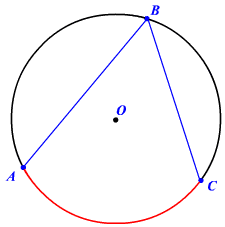Here, the circle with center $O$ has the inscribed angle $\angle ABC$. The other end points than the vertex, $A$ and $C$ define the intercepted arc $\stackrel{⌢}{AC}$ of the circle. The measure of $\stackrel{⌢}{AC}$  is the measure of its central angle. That is, the measure of $\angle AOC$.

## Inscribed Angle Theorem:

The measure of an inscribed angle is half the measure of the intercepted arc.

That is, $m\angle ABC=\frac{1}{2}m\angle AOC$.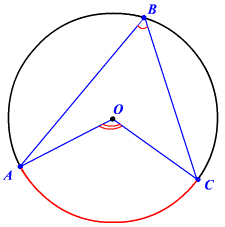This leads to the corollary that in a circle any two inscribed angles with the same intercepted arcs are congruent.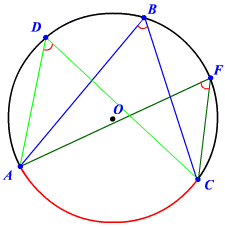Here, $\angle ADC\cong \angle ABC\cong \angle AFC$.

Example 1:

Find the measure of the inscribed angle $\angle PQR$.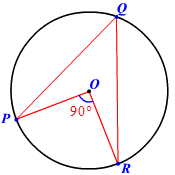By the inscribed angle theorem, the measure of an inscribed angle is half the measure of the intercepted arc.

The measure of the central angle $\angle POR$ of the intercepted arc $\stackrel{⌢}{PR}$ is $90°$.

Therefore,

$\begin{array}{l}m\angle PQR=\frac{1}{2}m\angle POR\\ \text{\hspace{0.17em}}\text{\hspace{0.17em}}\text{\hspace{0.17em}}\text{\hspace{0.17em}}\text{\hspace{0.17em}}\text{\hspace{0.17em}}\text{\hspace{0.17em}}\text{\hspace{0.17em}}\text{\hspace{0.17em}}\text{\hspace{0.17em}}\text{\hspace{0.17em}}\text{\hspace{0.17em}}\text{\hspace{0.17em}}\text{\hspace{0.17em}}\text{\hspace{0.17em}}\text{\hspace{0.17em}}\text{\hspace{0.17em}}\text{\hspace{0.17em}}\text{\hspace{0.17em}}=\frac{1}{2}\left(90°\right)\\ \text{\hspace{0.17em}}\text{\hspace{0.17em}}\text{\hspace{0.17em}}\text{\hspace{0.17em}}\text{\hspace{0.17em}}\text{\hspace{0.17em}}\text{\hspace{0.17em}}\text{\hspace{0.17em}}\text{\hspace{0.17em}}\text{\hspace{0.17em}}\text{\hspace{0.17em}}\text{\hspace{0.17em}}\text{\hspace{0.17em}}\text{\hspace{0.17em}}\text{\hspace{0.17em}}\text{\hspace{0.17em}}\text{\hspace{0.17em}}\text{\hspace{0.17em}}\text{\hspace{0.17em}}\text{\hspace{0.17em}}=45°\end{array}$.

Example 2:

Find $m\angle LPN$.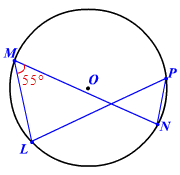In a circle, any two inscribed angles with the same intercepted arcs are congruent.

Here, the inscribed angles $\angle LMN$ and $\angle LPN$ have the same intercepted arc $\stackrel{⌢}{LN}$.

So, $\angle LMN\cong \angle LPN$.

Therefore, $m\angle LMN=m\angle LPN=55°$.

An especially interesting result of the Inscribed Angle Theorem is that an angle inscribed in a semi-circle is a right angle.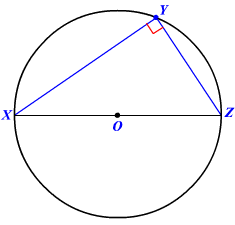In a semi-circle, the intercepted arc measures $180°$ and therefore any corresponding inscribed angle would measure half of it.